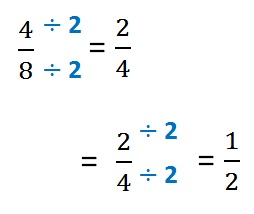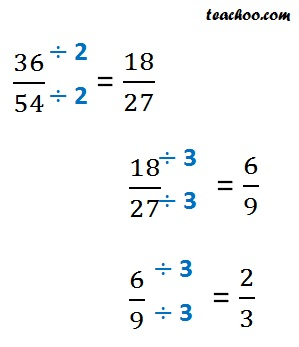Equivalent Fractions

Chapter 7 Class 6 Fractions
Concept wise

A fraction is simplest when numerator & denominator of a fraction can’t be smaller.

Example:- 2/4 can be simplified as 1/2

Let’s look at how to simplify fractions.

#### Simplify 4/8So, simplest from of 4/8 is 1/2

#### Simplify 36 / 54So, simplest from of 36/54 is 2/3

Get live Maths 1-on-1 Classs - Class 6 to 12

### Transcript

A fraction is simplest when numerator & denominator of a fraction can’t be smaller. Example:- 2/4 can be simplified as 1/2 Let’s look at how to simplify fractions. Simplify 𝟒/𝟖 4/8 = 2/4 So, simplest from of 4/8 is 1/2 Simplify 𝟑𝟔/𝟓𝟒 36/54 = 18/27 = 6/9 So, simplest from of 36/54 is 2/3